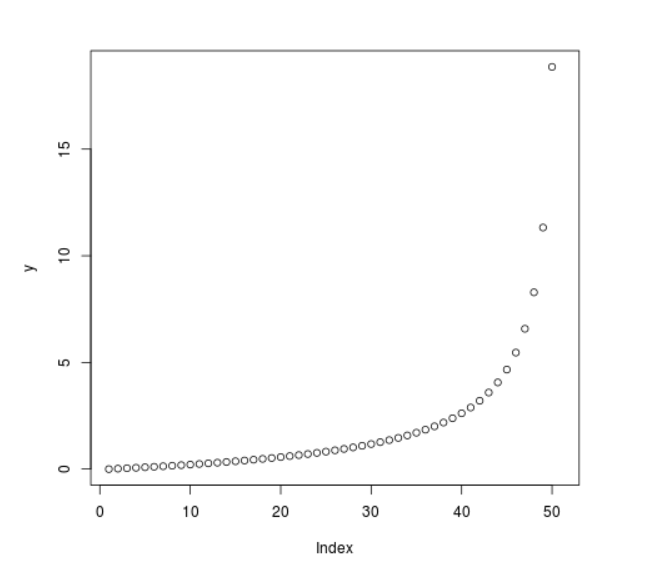# Compute the value of Quantile Function over F Distribution in R Programming – qf() Function

`qf()` function in R Language is used to compute the value of quantile function over F distribution for a sequence of numeric values. It also creates a density plot of quantile function over F Distribution.

Syntax: qf(x, df1, df2)

Parameters:
x: Numeric Vector
df: Degree of Freedom

Example 1:

 `# R Program to compute value of ` `# Quantile Function over F Distribution ` ` `  `# Creating a sequence of x-values ` `x <``-` `seq(``0``, ``1``, by ``=` `0.2``) ` ` `  `# Calling qf() Function ` `y <``-` `qf(x, df1 ``=` `2``, df2 ``=` `3``) ` `y `

Output:

``` 0.0000000 0.2405958 0.6085817 1.2630236 2.8860266       Inf
```

Example 2:

 `# R Program to compute the value of ` `# Quantile Function over F Distribution ` ` `  `# Creating a sequence of x-values ` `x <``-` `seq(``0``, ``1``, by ``=` `0.02``) ` ` `  `# Calling qf() Function ` `y <``-` `qf(x, df1 ``=` `2``, df2 ``=` `3``) ` ` `  `# Plot a graph ` `plot(y) `

Output:Whether you're preparing for your first job interview or aiming to upskill in this ever-evolving tech landscape, GeeksforGeeks Courses are your key to success. We provide top-quality content at affordable prices, all geared towards accelerating your growth in a time-bound manner. Join the millions we've already empowered, and we're here to do the same for you. Don't miss out - check it out now!